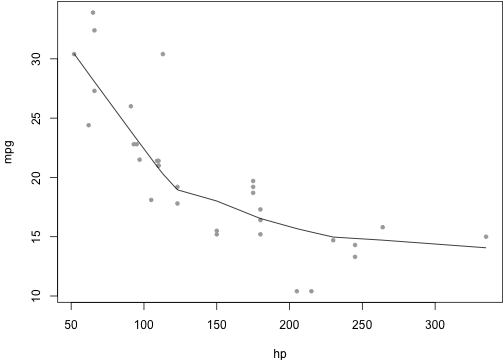martes, 30 de abril de 2013

Knitr en R

A Minimal Example for Markdown <!-- Styles for R syntax highlighter

A Minimal Example for Markdown

This is a minimal example of using knitr to produce an HTML page from Markdown.

R code chunks

# set global chunk options: images will be 7x5 inches
opts_chunk\$set(fig.width = 7, fig.height = 5)
Now we write some code chunks in this markdown file:
x <- 1 + 1  # a simple calculator
set.seed(123)
rnorm(5)  # boring random numbers
##  -0.56048 -0.23018  1.55871  0.07051  0.12929
We can also produce plots:
par(mar = c(4, 4, 0.1, 0.1))
with(mtcars, {
plot(mpg ~ hp, pch = 20, col = "darkgray")
lines(lowess(hp, mpg))
})Inline code

Inline R code is also supported, e.g. the value of x is 2, and 2 × π = 6.2832.

Math

LaTeX math as usual: $$f(\alpha, \beta) \propto x^{\alpha-1}(1-x)^{\beta-1}$$.

Misc

You can indent code chunks so they can nest within other environments such as lists.
1. the area of a circle with radius x
pi * x^2

##  12.57

2. OK, that is great
To compile me, use
library(knitr)
knit("knitr-minimal.Rmd")

Conclusion

Markdown is super easy to write. Go to knitr homepage for details
.
-->